NEET  >  Qualitative & Quantitative Analysis of Organic Compounds

# Qualitative & Quantitative Analysis of Organic Compounds - Notes | Study Chemistry Class 11 - NEET

 Table of contentsWhat is Qualitative Analysis?Estimation of HalogensTypes of Organic Reactions1 Crore+ students have signed up on EduRev. Have you?

What is Qualitative Analysis?

Quantitative analysis is an analysis method used to determine the number of elements or molecules produced during a chemical reaction. Organic compounds are comprised of carbon, hydrogen, oxygen, nitrogen, phosphorus, sulphur and halogens. The various methods used for the measurement of percentage composition of elements in an organic compound are explained here.
Qualitative analysis is the analysis of the species present in a given compound. For example, if a compound is taken, the qualitative analysis would be more focused on finding the elements and the ions present in the compound rather than study as to how much they are present.

Detection of C and H

C and H are detected by heating the compound with CuO in a dry test tube. They are oxidised to CO2 and H2O respectively. If the CO2 turns lime water milky, and H2O turns anhydrous CuSO4 blue, then the presence of C and H is confirmed.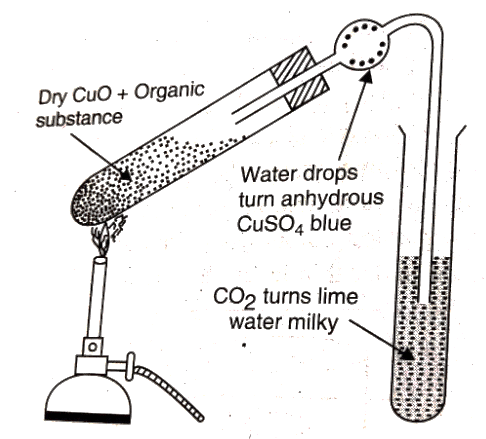Detection of Carbon and Hydrogen

Test for Phosphorous

The organic compound is heated with an oxidising agent to oxidise phosphorous to phosphate. The solution is then boiled with concentrated HNO3 and treated with ammonium molybdate. Yellow precipitate confirms the presence of phosphorous.
The reaction is given below,
Na3PO4 + 3HNO3 → H3PO4 + 3NaNO3
H3PO4 + 12(NH4)2MoO4 + 21HNO3 → (NH4)3PO4.12MoO3 + 21NH4NO3 + 12H2O
Quantitative analysis is more towards finding out how much of the elements are present, that is, their amounts.

Estimation of C and H

Liebig’s Combustion Method

A known mass of the compound is heated with CuO. The carbon present is oxidised to CO2 and hydrogen to H2O. The CO2 is absorbed in KOH solution, while H2O is absorbed by anhydrous CaCl2 and they are weighed.
Percentage of C = 12/44 x ((Mass of CO2)/(Mass of compound)) x 100
Percentage of H = 2/18 x ((Mass of H2O)/(Mass of compound)) x 100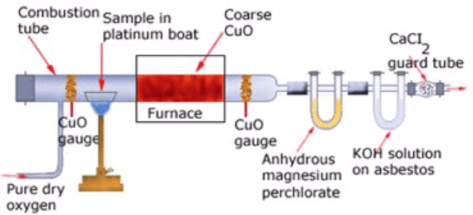Liebig’s combustion method

Estimation of Halogens

Carius Method

A known mass of the compound is heated with Conc. HNO3 in the presence of AgNO3 in a hard glass tube called Carius tube. C and H are oxidised to CO2 and H2O. The halogen forms the corresponding AgX. It is filtered, dried and weighed.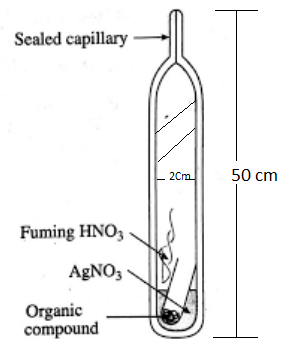Estimation of Halogens by Carius method

Percentage of X = ((Atomic mass of X)/(Molecular mass of AgX))x((Mass of AgX)/(Mass of the compound))x100

Calculations:

Let the mass of the given organic compound be m g.
Suppose the mass of AgX formed = m1 g.
We know that 1 mol of AgX consists of 1 mol of X.
So, in m1 g of AgX , mass of halogen =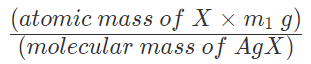Percentage of halogen =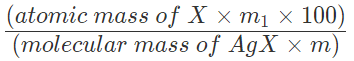Estimation of Sulphur

A known mass of the compound is heated with conc. HNO3 in the presence of BaCl2 solution in Carius tube. Sulphur is oxidised to H2SO4 and precipitated as BaSO4. It is then dried and weighed.
Percentage of S= ((Atomic mass of S)/(Molecular mass of BaSO4)) x (( Mass of BaSO4)/(Mass of the compound)) x 100

Calculations:

Suppose the mass of organic compound = mg
Let the mass of barium sulphate formed = m1 g
We know that 32 g sulphur is present in 1 mol of BaSO4
Therefore, 233 g BaSO4 contains 32 g sulphur:
⇒ M1 g of BaSO4 contains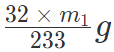of sulphur
Percentage of sulphur =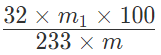Estimation of Phosphorus

A known mass of the compound is heated with HNO3 in a Carius tube, which oxidises phosphorous to phosphoric acid. It is then precipitated as ammonium phosphomolybdate ((NH4)3PO4.12MoO3) by adding NH3 and ammonium molybdate ((NH4)2MoO4). It is filtered, dried and weighed.
Percentage of P =
((Atomic mass of P)/(Molecular mass of (NH4)3PO4.12MoO3)) x ((Mass of (NH4)3PO4.12MoO3)/(Mass of compound)) x 100

Estimation of Nitrogen

Estimation of Nitrogen by Dumas Method

A known mass of the compound is heated with CuO in an atmosphere of CO2, which yields free nitrogen along with CO2 and H2O.
CxHyNz + (2x+ 0.5y) CuO → xCO2 + 0.5y H2O + 0.5z (N2) + (2x+ 0.5y)Cu
The gases are passed over a hot copper gauze to convert trace amounts of nitrogen oxides to N2. The gaseous mixture is collected over a solution of KOH which absorbs CO2, and nitrogen is collected in the upper part of the graduated tube.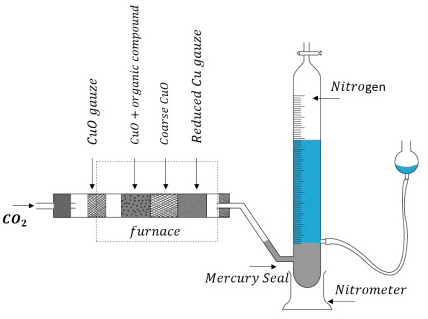Estimation of Nitrogen by Dumas Method

Let the volume of N2 collected be V1 mL.
Then, volume of N2 at STP = (P1V1 x 273)/ (760 x T1) = V mL
Where P1 and V1 are the pressure and volume of N2.
P1 = Atmospheric pressure – aqueous tension
22.4 L of N2 weighs 28 g,
Therefore, V ml of N2 weighs
= (28 x V)/22400 grams
Percentage of N would be,
= (28/22400) x (V/ Mass of compound) x 100

Estimation of Nitrogen by Kjeldahl Method

A known mass of an organic compound is (0.5 g) is mixed with K2SO4 (10 g) and CuSO4 (1.0 g) and conc.H2SO4 (25 mL), and heated in a Kjeldahl’s flask.
CuSO4 acts as a catalyst, while K2SO4 raises the boiling point of sulphuric acid. The nitrogen in the compound is quantitatively converted to (NH4)2SO4. The resulting mixture is reacted with an excess of NaOH solution and the NH3 so evolved, is passed into a known but the excess volume of standard acid.
The acid left unreacted is estimated by titration with some standard alkali. Thus the percentage of nitrogen can be calculated.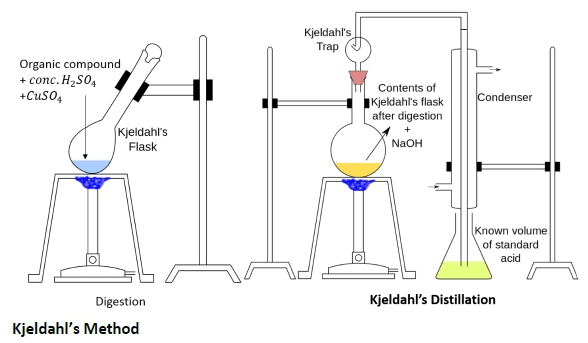Estimation of Nitrogen by Kjeldahl Method

The reactions are given below:

• C + H + S → CO2 + H2O + SO2
• N → (NH4)2SO4
• (NH4)2SO4 + 2NaOH → Na2SO4 + 2NH3 + 2H2O
• 2NH3 + H2SO4 → (NH4)2SO4

Calculation of the percentage of N
Let the mass of the organic compound be mg.
Volume of H2SO4 (Molarity M) = V mL
The volume of NaOH of molarity M used for titration excess of H2SO4= V1 mL
mEq of excess H2SO4 = mEq of NaOH
= MV1 mEq
Total mEq of H2SO4 taken = 2MV
mEq of H2SO4 used for neutralisation of NH3 = (2MV – MV1)
Therefore,
mEq of NH3 = (2MV-MV1)
1000 mEq or 1000 mL of NH3 solution contains = 17 g of NH3 (or) 14 g of N
Therefore,
(2MV-MV1) mEq of NH3 contains
=(14 x (2MV-MV1)) / 1000 g of N
Percentage of N = ((14x(2MV - MV1)) x (100/(1000 x m)))

Estimation of Oxygen – Aluise’s method

A known mass of the compound is decomposed by heating it in the presence of N2 gas. The mixture of gases so produced is passed over red hot coke. This is done so that all the O2 is converted to CO. This mixture is heated with I2O5 in which CO is oxidised to CO2 liberating I2.
The reactions are given below,
Organic compound → Other gaseous products + O2
2C + O2 → 2CO
I2O5 + 5CO → 5CO2 + I2
Percentage of O = ((Molecular mass of O2/Molecular mass of CO2) x (Mass of CO2/Mass of the compound) x 100

Types of Organic Reactions

Organic reactions are the chemical reactions that are undergone by organic compounds (the chemical compounds containing carbon). A few important types of organic reactions are illustrated below.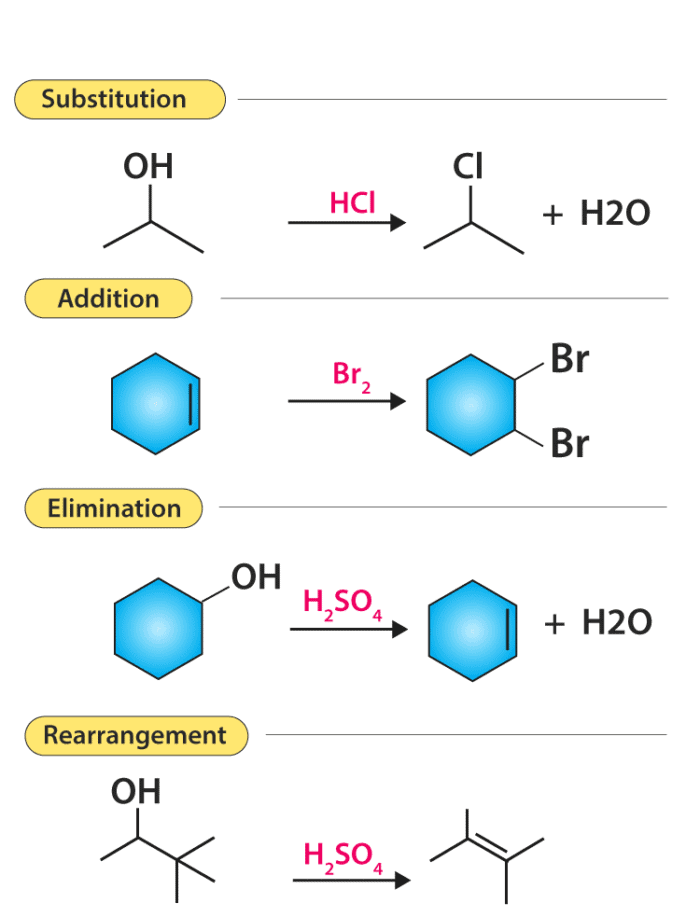There are mainly five types of organic reactions:

• Substitution reaction
• Elimination reaction
• Oxidation-Reduction Reactions.

Substitution Reactions

In a substitution reaction, one atom or a group of atoms is substituted by another atom or a group of atoms to form a new substance. Let us take an example of a C-Cl bond, in which the carbon atom has a partial positive charge due to the presence of highly electronegative chlorine atom. In a nucleophilic substitution reaction, the nucleophile must have a pair of electrons and also should have a high affinity for the electropositive species as compared to the substituent which was originally present.

Elimination reaction

There are some reactions which involve the elimination or removal of the adjacent atoms. After these multiple bonds are formed and there is a release of small molecules as products. One of the examples of elimination reaction is the conversion of ethyl chloride to ethylene.
CH3CH2Cl →   CH2=CH2 + HCl
In the above reaction, the eliminated molecule is HCl, which is formed by the combination of  H+ from the carbon atom which is on the left side and Cl– from the carbon atom which is on the right side.

Addition reaction is nothing but just the opposite of elimination reaction. In an addition reaction, the components A and B are added to the carbon-carbon multiple bonds and this is called addition reaction. In the reaction given below when HCl is added to ethylene, it will give us ethylene chloride.
HCl + CH2=CH→ CH3CH2Cl

Many of the organic reactions involve radicals. Addition of a halogen to a   saturated hydrocarbon involves free radical mechanism. There are three stages involved in a radical reaction i.e. initiation, propagation, and termination. Initially when the weak bond is broken initiation of the reaction takes place with the formation of free radicals. After that when the halogen is added to the hydrocarbon a radical is produced and finally, it gives alkyl halide.

The document Qualitative & Quantitative Analysis of Organic Compounds - Notes | Study Chemistry Class 11 - NEET is a part of the NEET Course Chemistry Class 11.
All you need of NEET at this link: NEET

## Chemistry Class 11

204 videos|331 docs|229 tests

## Chemistry Class 11

204 videos|331 docs|229 tests

### How to Prepare for NEET

Read our guide to prepare for NEET which is created by Toppers & the best Teachers

Track your progress, build streaks, highlight & save important lessons and more!

,

,

,

,

,

,

,

,

,

,

,

,

,

,

,

,

,

,

,

,

,

;